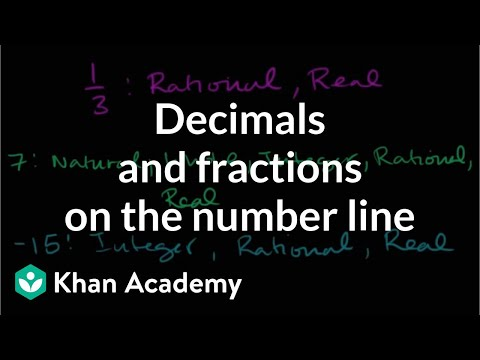Video

# Missing numbers on the number line examples (Full video)

Description: Given a few negative numbers on a number line, let's see if we can determine what negative numbers are elsewhere on the number line. Created by Sal Khan. We have three different number lines here, and on each number line they mark off a couple of these marks, this is negative two this is negative 10, negative five, negative 11, and then we need to figure out what the blue dot represents. Try to figure out what number does this blue dot represent on these different number lines? So we're gonna go where they gave us this mark is negative two, this is negative 10, and we need to figure out this blue one that's further to the left of negative 10.

### Other videos you might be interested in### Area of a parallelogram on the coordinate plane (Full video)